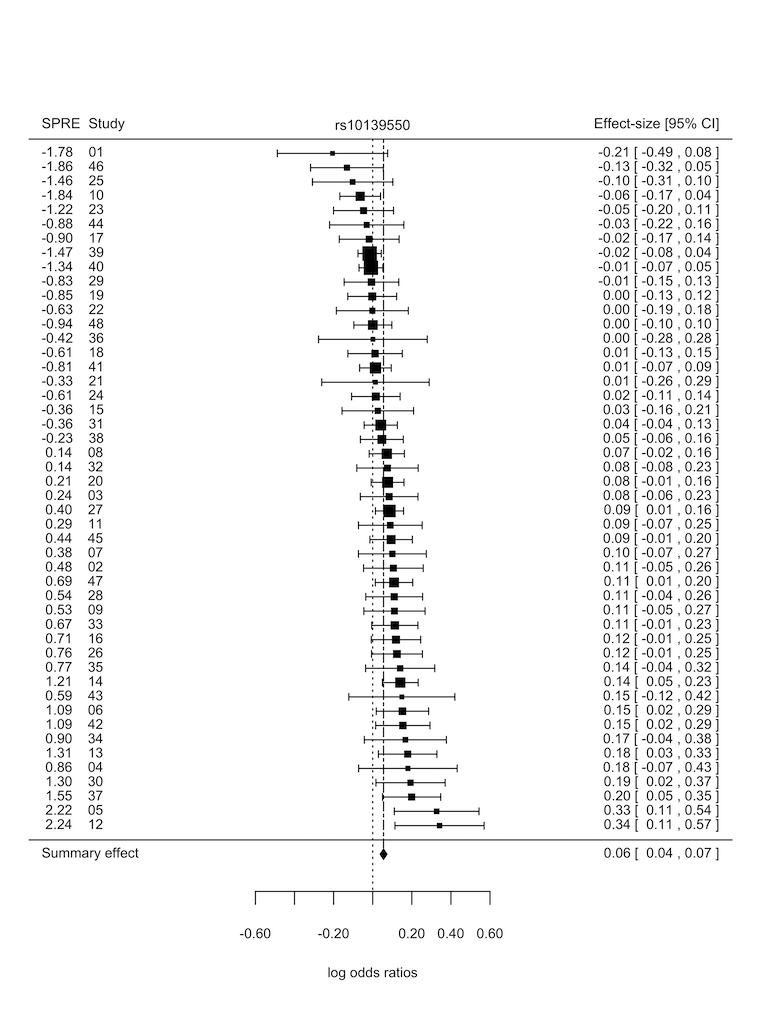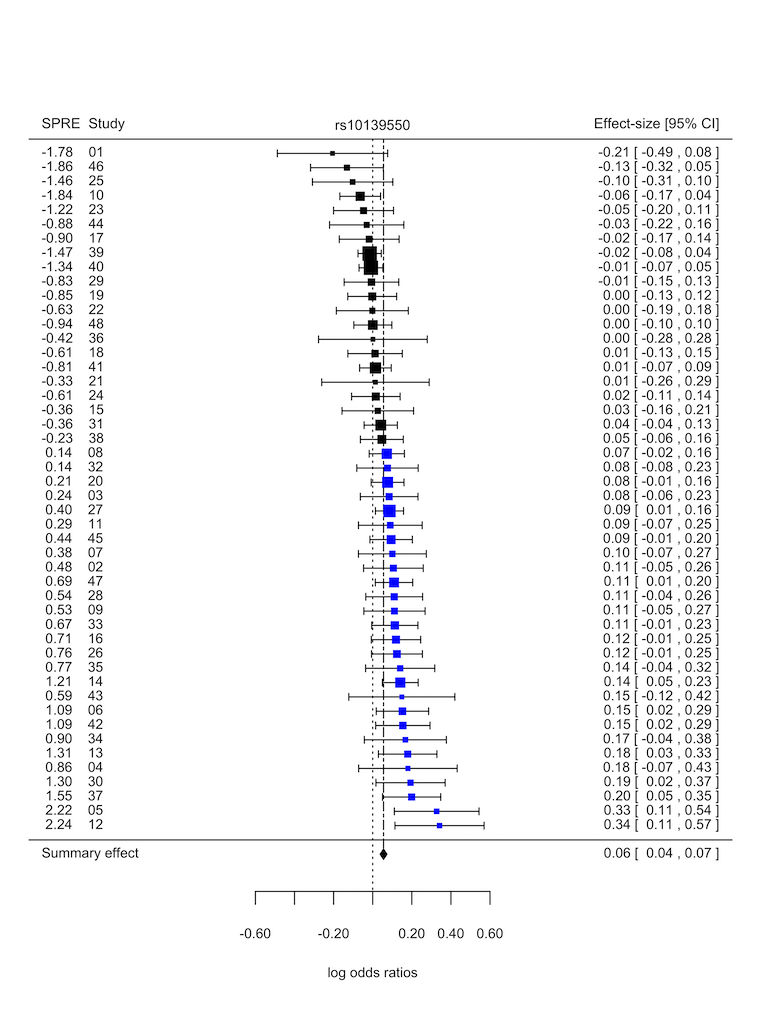## Tutorial Goals

1. Outline importance of identifying overly influential outliers in meta-analysis.

2. Demonstrate how the getspres R package can be used to identify outlier studies showing extreme effects in meta-analyses.

## It's important to check for potential outliers when performing meta-analysis, here's why

• The presence of outlier studies showing outsized effects in a meta-analysis might contribute to inflated genetic signals yielding false positive or negative genetic associations.

• Heterogeneity sources which might contribute to overly influential outliers in genetic association meta-analysis include: population structure and genotyping error.

## Two popular approaches for identifying overly influential outliers

• Outlier studies showing extreme effects can be identified quantitatively through the calculation of SPRE statistics (standardised predicted random-effects) or visually via forest plots.

• Forest plots illustrate the distribution of genetic effect estimates reported by studies in a meta-analysis.

• SPRE statistics are precision-weighted residuals that summarise the direction and extent with which genetic effects reported by participating studies in a meta-analysis deviate from the summary or average genetic effect. Another term commonly used when referring to SPRE statistics is internally studentized residuals. Detailed statistical theory on SPRE statistics can obtained from the following references:

1. Harbord, R. M., & Higgins, J. P. T. (2008). Meta-regression in Stata. Stata Journal 8: 493‚Äì519.

2. Magosi LE, Goel A, Hopewell JC, Farrall M, on behalf of the CARDIoGRAMplusC4D Consortium (2017) Identifying systematic heterogeneity patterns in genetic association meta-analysis studies. PLoS Genet 13(5): e1006755. https://doi.org/10.1371/journal.pgen.1006755.

3. Lerato E Magosi, Anuj Goel, Jemma C Hopewell, Martin Farrall, on behalf of the CARDIoGRAMplusC4D Consortium, Identifying small-effect genetic associations overlooked by the conventional fixed-effect model in a large-scale meta-analysis of coronary artery disease, Bioinformatics, , btz590, https://doi-org.ezp-prod1.hul.harvard.edu/10.1093/bioinformatics/btz590

## The getspres R package: A two for one bargain in outlier diagnostics!

• The getspres R package combines calculation of SPRE statistics and generation of forest plots in a single tool, making it easier to identify overly influential outliers with effects that differ substantially from those reported by other studies in a meta-analysis.

• The getspres package comprises 2 functions:

• getspres: calculates SPRE statistics
• plotspres: generates forest plots showing SPRE statistics

## Let's take a look at some examples:

### Data: heartgenes214

To explore heterogeneity with the getspres R package, we shall use the `heartgenes214` dataset.

The `heartgenes214` dataset is a case-control meta-analysis of coronary artery disease.

The `heartgenes214` dataset is documented in ?heartgenes214'. It comprises summary data (effect-sizes and corresponding standard errors) for 48 studies (68,801 cases and 123,504 controls), at 214 lead variants independently associated with coronary artery disease (P < 0.00005, FDR < 5%). Of the 214 lead variants, 44 are genome-wide significant (P < 5e-08). The meta-analysis dataset is based on individuals from six ancestry groups, namely: African American, Hispanic American, East Asian, South Asian, Middle Eastern and European.

#### The data was sourced from:

Magosi LE, Goel A, Hopewell JC, Farrall M, on behalf of the CARDIoGRAMplusC4D Consortium (2017) Identifying systematic heterogeneity patterns in genetic association meta-analysis studies. PLoS Genet 13(5): e1006755. https://doi.org/10.1371/journal.pgen.1006755.

``````
# Load libraries and inspect data  ------------------------------------

library(getspres)

# Exploring heterogeneity at 3 variants in heartgenes214

head(heartgenes214)

str(heartgenes214)

heartgenes3 <- subset(heartgenes214,
variants %in% c("rs10139550", "rs10168194", "rs11191416"))

# Exploring the `getspres` and `plotspres` functions

?getspres

?plotspres

# Calculating SPRE statistics  -----------------------------------

getspres_results <- getspres(beta_in = heartgenes3\$beta_flipped,
se_in = heartgenes3\$gcse,
study_names_in = heartgenes3\$studies,
variant_names_in = heartgenes3\$variants)

# Explore results generated by the getspres function
str(getspres_results)

# Retrieve number of studies and variants
getspres_results\$number_variants
getspres_results\$number_studies

# Retrieve SPRE dataset
df_spres <- getspres_results\$spre_dataset
head(df_spres)

# Extract SPREs from SPRE dataset
head(spres <- df_spres[, "spre"])

# Exploring available options in the getspres function:

#     1. Estimate heterogeneity using "REML", default is "DL"
#     2. Calculate SPRE statistics verbosely

getspres_results <- getspres(beta_in = heartgenes3\$beta_flipped,
se_in = heartgenes3\$gcse,
study_names_in = heartgenes3\$studies,
variant_names_in = heartgenes3\$variants,
tau2_method = "REML",
verbose_output = TRUE)

# Generating forest plots  ---------------------------------------

# Forest plot with default settings
# Tip: To store plots set save_plot = TRUE (useful when generating multiple plots)
plotspres_res <- plotspres(beta_in = df_spres\$beta,
se_in = df_spres\$se,
study_names_in = as.character(df_spres\$study_names),
variant_names_in = as.character(df_spres\$variant_names),
spres_in = df_spres\$spre,
save_plot = TRUE)

# Explore results generated by the plotspres function

# Retrieve number of studies and variants
plotspres_res\$number_variants
plotspres_res\$number_studies

# Retrieve fixed and random-effects meta-analysis results
fixed_effect_res <- plotspres_res\$fixed_effect_results
random_effects_res <- plotspres_res\$random_effects_results

# Retrieve dataset that was used to generate forest plots
df_plotspres <- plotspres_res\$spre_forestplot_dataset

# Retrieve more detailed meta-analysis output
str(plotspres_res)

````````````
# Explore available options for plotspres forest plots:
#   1. Colorize study-effect estimates according to SPRE statistic values
#   2. Label studies by study number instead of study names
#   3. Format study labels (useful when using study numbers as study labels)
#   4. Change text size
#   5. Adjust x and y axes limits
#   6. Change method used to estimate amount of heterogeneity from "DL" to "REML"
#   7. Run verbosely to show intermediate results
#   8. Adjust label (i.e. column header) positions
#   9. Save plot as a tiff file (useful when generating multiple plots)

# Colorize study-effect estimates according to SPRE statistic values

# Use a dual colour palette for observed study effects so that study effect estimates
#   with negative SPRE statistics are coloured differently from those with positive
#   SPRE statistics.
plotspres_res <- plotspres(beta_in = df_spres\$beta,
se_in = df_spres\$se,
study_names_in = as.character(df_spres\$study_names),
variant_names_in = as.character(df_spres\$variant_names),
spres_in = df_spres\$spre,
spre_colour_palette = c("dual_colour", c("blue","black")),
save_plot = TRUE)

````````````

# Use a multi-colour palette for observed study effects so that study effects estimates
#   are colored in a gradient according to SPRE statistic values.
#   Available multi-colour palettes:
#
#       gr_devices_palettes: "rainbow", "cm.colors", "topo.colors", "terrain.colors"
#                            and "heat.colors"
#
#       colorspace_hcl_hsv_palettes: "rainbow_hcl", "diverge_hcl", "terrain_hcl",
#                                    "sequential_hcl" and "diverge_hsl"
#
#       color_ramps_palettes: "matlab.like", "matlab.like2", "magenta2green",
#                             "cyan2yellow", "blue2yellow", "green2red",
#                             "blue2green" and "blue2red"

plotspres_res <- plotspres(beta_in = df_spres\$beta,
se_in = df_spres\$se,
study_names_in = as.character(df_spres\$study_names),
variant_names_in = as.character(df_spres\$variant_names),
spres_in = df_spres\$spre,
spre_colour_palette = c("multi_colour", "rainbow"),
save_plot = TRUE)

``````